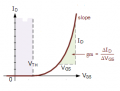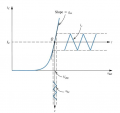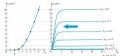# Why transconductance(gm) of a MOSFET is expressed with two different ways?

#### Mustafa Uzuner

Joined Dec 17, 2016
10
Hi,

I am trying to understand the transconductance of the mosfets. By definition it is the change in the drain current divided by the gate-source voltage. However, some sources shows it with a derivative equation such as:

But sometimes it is shown without the derivative operation.

Some of the forums I found says that derivative is not used in the case of a small signal analysis. But it doesn't make sense to me and also Razavi's Design of Analog CMOS Integrated Design shows this equation with derivative in the case of a small signal analysis. So, is there anyone to clarify this? Thanks for your help.

#### Papabravo

Joined Feb 24, 2006
20,160
I think both approaches are correct under different sets of assumptions. In the first instance you are getting the instantaneous value of gm at a particular point on the characteristic curve. In the second case you are getting an average value of the ratio over some portion of the characteristic curve. I see no conflict here. Why do you see a problem?

Another question to ask yourself is: "what is the shape of the characteristic?"
1. Over a small interval
2. Over a large interval
In your design, what would you do if gm was a normally distributed random variable with a mean of μ and a variance of σ²

Last edited:

#### Mustafa Uzuner

Joined Dec 17, 2016
10
The problem is I can't decide which one to use during an analysis of a circuit. When I read your post I thought the second approach is more appropriate cause mostly I am interested in a general characteristics rather than a specific time. However, first approach is used quite frequently in the textbooks.

#### Papabravo

Joined Feb 24, 2006
20,160
The problem is I can't decide which one to use during an analysis of a circuit. When I read your post I thought the second approach is more appropriate cause mostly I am interested in a general characteristics rather than a specific time. However, first approach is used quite frequently in the textbooks.
I was trying to make a subtle point, that good designs are not dependent on a particular value of a device parameter. From the datasheet you can often find a typical or mean value and at least one extreme value which gives you an estimate of the standard deviation, σ. The particular value of a device parameter just should not matter,

#### LvW

Joined Jun 13, 2013
1,647
Hi,

I am trying to understand the transconductance of the mosfets. By definition it is the change in the drain current divided by the gate-source voltage. However, some sources shows it with a derivative equation such as:

But sometimes it is shown without the derivative operation.

Some of the forums I found says that derivative is not used in the case of a small signal analysis. But it doesn't make sense to me and also Razavi's Design of Analog CMOS Integrated Design shows this equation with derivative in the case of a small signal analysis. So, is there anyone to clarify this? Thanks for your help.
* The first expression is, of course, correct because it shows the DEFINITION of the transconductance gm.
* However, I think, the second expression is not correct. Where does it come from? I could not find this expression in Razavis book.
This expression would lead to an INFINITE transconduchtance for VGS=0 - which is totally wrong
A similar expression (Ic/Vbe) is valid only for the BJT because the function Ic=f(Vbe) is an exponential one !
gm=(2/Vth)SQRT(Ids*Id)
(with theshold voltage Vth and Ids=current Id for Vgs=0 )

Answer Papabravo: "The particular value of a device parameter just should not matter, "
I rather think - The tansconductance gm of a transistor is the ONLY parameter which matters as far as the voltage gain of a stage is concerned.

Last edited:

#### Wolframore

Joined Jan 21, 2019
2,590
For small signal analysis (very small) the equation is simplified to just the linear term.

•GMKF

#### crutschow

Joined Mar 14, 2008
32,073
For small signal analysis (very small) the equation is simplified to just the linear term.
Yes, the second term only really applies to a small signal analysis (where the MOSFET is modeled as a linear gain).
If you just calculated the DC (large signal) value of Id/Vgs at some particular bias point, you would not get a valid value for gm since Vgs would include whatever the Vgh(thres) of the MOSFET is.

•GMKF

#### MrAl

Joined Jun 17, 2014
10,096
Hi,

This is what happens when a formula is taken out of context...it can not always be understood.
In physics there is really no such thing as an arbitrary formula, it is always associated with something physical like a circuit and method.

#### Papabravo

Joined Feb 24, 2006
20,160
Another word about device parameters. The whole reason that the operational amplifier was such an important development is EXACTLY because you could build an amplifier that did not depend on device characteristics. The stage depended on passive components whose values could be made with tighter tolerance than any vacuum tube or semiconductor of that era. Why is this the case? It is primarily because the op amp is operated at a gain that is much smaller than it's open loop gain. As long as the transconductance falls within a range to a 99% confidence we can design usable amplifiers that do not depend on a specific value of gm, until the cows come home.

#### LvW

Joined Jun 13, 2013
1,647
............ As long as the transconductance falls within a range to a 99% confidence we can design usable amplifiers that do not depend on a specific value of gm, until the cows come home.
Yes - agreed. But Mustafa U. was asking for the transconductance of a single transistor. And - as we know - the transconductance gm of a FET is much smaller if compared with the BJT. I think, we cannot neglect it for a single stage amplifier.

#### Wolframore

Joined Jan 21, 2019
2,590
I believe we need to go a little further to explain the reason why we ignore the full calculation for our FET In small signal analysis. I found this conductance curve:for small signal analysis we are interested in utilizing the small linear area. This Is similar for diodes and BJTs. By biasing our AC in the linear area (Q) we can ignore the non linear curve utilize the device as a linear amplifier. Like this (sorry this one is a BJT curve but very similar).while the derivative is correct when utilizing the MOSFET in the linear area we simplify the calculation just as we do with BJTs and bias the signal into a linear area and setting the quiescent point.

#### LvW

Joined Jun 13, 2013
1,647
I believe we need to go a little further to explain the reason why we ignore the full calculation for our FET In small signal analysis. I found this conductance curve:
.........................
for small signal analysis we are interested in utilizing the small linear area. This Is similar for diodes and BJTs. By biasing our AC in the linear area (Q) we can ignore the non linear curve utilize the device as a linear amplifier. Like this (sorry this one is a BJT curve but very similar).
.........................
While the derivative is correct when utilizing the MOSFET in the linear area we simplify the calculation just as we do with BJTs and bias the signal into a linear area and setting the quiescent point.
Yes - I agree to everything - as far as the "linearity" is concerned. But this was not the question - I think.
The question was if the expression which is the DEFINITION of the FETs transconductance would give the same transconductance value as the simple form gm=Id/Vgs. And this is NOT the case.
Of course, the transconductance definition is identical for BJTs and FETs - however, the function which is used to calculate the slope (identical to the transconductance) is not "very similar" but quite different (exponential vs. quadratic).
Hence, if we wish to express the transconductance using the quiescent current Ic resp. Id, we get two different expressions (see post #5).

#### MrAl

Joined Jun 17, 2014
10,096
Hi again,

The short answer i think is that one uses direct DC quantities to calculate the gm and the other uses small AC quantities to calculate the gm.

But to add to the confusion, there is another definition:
gm=2*Id/(Vg-Vth)
where
Id is the drain current,
Vg is the DC gate voltage,
Vth is the threshold voltage.
I found something like this to work a long time ago (might not be exactly that though) when i was not aware of that expression. It comes from a particular model for the MOSFET.
I based my derivation on the simple fact that the device has little response until we reach Vth.

#### LvW

Joined Jun 13, 2013
1,647
But to add to the confusion, there is another definition:
gm=2*Id/(Vg-Vth)
where
Id is the drain current,
Vg is the DC gate voltage,
Vth is the threshold voltage.
Hi - MrAl, the expression for gm as mentioned in my post#5 is nothing else than the slope of the quadratic Id=f(Vgs) function: Id=Id,s(1-Vgs/Vth)².

#### MrAl

Joined Jun 17, 2014
10,096
Hi,

You mean the 2*Id/(Vg-Vth) one?
That comes from a particular model of the MOSFET and constant Vds and a Taylor series where when we assume small changes the terms above linear are left out as usual.
I did not derive that one exactly though i'd have to look that up. The one i derived is different i think but i am trying to remember what it was and how i derived it. I have to look through some notes or just try to remember how i came about it. I'll do some searching.

#### LvW

Joined Jun 13, 2013
1,647
MrAl - Thank you for this information.

#### MrAl

Joined Jun 17, 2014
10,096
Oh you're welcome i just wish i could remember the other one too.

#### Jony130

Joined Feb 17, 2009
5,445
The drain current of a MOSFET is usually expressed like this:

Id = Kp(Vgs- Vt)^2 thus, gm = 2Kp(Vgs - Vt) but if we substitute Kp by Kp = Id/(Vgs - Vt)^2 we will end with:

gm = 2 * Id/(Vgs - Vt)^2 * (Vgs - Vt) = 2Id/(Vgs- Vt)

gm = 2Id/(Vgs- Vt)

#### Mustafa Uzuner

Joined Dec 17, 2016
10
* The first expression is, of course, correct because it shows the DEFINITION of the transconductance gm.
* However, I think, the second expression is not correct. Where does it come from? I could not find this expression in Razavis book.
This expression would lead to an INFINITE transconduchtance for VGS=0 - which is totally wrong
A similar expression (Ic/Vbe) is valid only for the BJT because the function Ic=f(Vbe) is an exponential one !
gm=(2/Vth)SQRT(Ids*Id)
(with theshold voltage Vth and Ids=current Id for Vgs=0 )

Answer Papabravo: "The particular value of a device parameter just should not matter, "
I rather think - The tansconductance gm of a transistor is the ONLY parameter which matters as far as the voltage gain of a stage is concerned.
Hi, you can find the second expression in some sources such as: http://atlas.physics.arizona.edu/~s...S2017/LectureSupplements/Transconductance.pdf
This definition is mostly done for small signal analysis. I am using Razavi's book as a reference for my Analog IC course and as you said and as far as I know, he never uses it.

Wolframore said in this first answer
"For small signal analysis (very small) the equation is simplified to just the linear term."

Maybe MOS circuits has a specific behavior in very small signal analysis wihch I don't know.

#### WBahn

Joined Mar 31, 2012
28,502
* The first expression is, of course, correct because it shows the DEFINITION of the transconductance gm.
* However, I think, the second expression is not correct. Where does it come from? I could not find this expression in Razavi`s book.
This expression would lead to an INFINITE transconduchtance for VGS=0 - which is totally wrong
A similar expression (Ic/Vbe) is valid only for the BJT because the function Ic=f(Vbe) is an exponential one !
gm=(2/Vth)SQRT(Ids*Id)
(with theshold voltage Vth and Ids=current Id for Vgs=0 )
The small-signal transconductance of a BJT is NOT given by Ic/Ibe.

$$gm \; = \; \frac{dI_c}{dV_{BE}} \; = \; \frac{d}{dV_{BE}} I_S \, e^{\frac{V_{BE}}{V_T}} \; = \; \frac{1}{V_T} I_S \, e^{\frac{V_{BE}}{V_T}} \; = \; \frac{I_C}{V_T}$$

Note that this is assuming an ideality factor of 1.

For a MOSFET in the saturation region (and ignoring the channel length modulation) you have

$$gm \; = \; \frac{dI_D}{dV_{GS}} \; = \; \frac{d}{dV_{GS}} K \, {\left( V_{GS} \, - \, V_{th} \right)}^2 \; = \; 2K \, {\left( V_{GS} \, - \, V_{th} \right)} \; = \; \frac{2K{\left( V_{GS} \, - \, V_{th} \right)}^2}{\left( V_{GS} \, - \, V_{th} \right)} \; = \; \frac{2I_D}{\left( V_{GS} \, - \, V_{th} \right)}$$

The term (Vgs - Vth) is often referred to as Vod, the overdrive voltage.